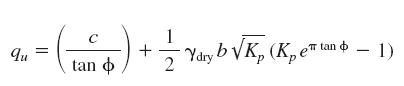Awarded as the best online publication by CIDC

# Soil Engineering

Describe Compaction Equipment Rollers

Soil compaction is done by Rollers. Many models of these rollers can be found in the market. Depending on the soil type and conditions, we chose rollers to work on them. For example, for soils with high % of clay we mainly use Sheepsfoot rollers and for granular soils we use vibrating rollers. The selection of roller depends on other factors like speed, desired compaction etc

The table below shows the average speeds, mi/h (km/h) under normal conditions:

 Type mi/h km/h Grid rollers 12 19.3 Sheepsfoot rollers 3 4.8 Tamping rollers 10 16.1 Pneumatic rollers 8 12.8

To calculate compaction production use the following formula
yd3/h (m3/h) = 16WSLFE/P
where
W = width of roller, ft (m)
P = number of passes
S = roller speed, mi/h (km / h)
L = lift thickness, in (mm)
F = ratio of pay yd3 ( m3) to loose yd3 ( m3)
E = efficiency factor for excellent =0.90, average=0.80, poor= 0.75

Soil Compaction Tests

There are many types of Soil compaction tests which are performed on soil. Some of these are :-

1) The Sand Cone Method
One of the most common test to determine the field density of soil is the sand-cone method. But it has a major limitation that this test is not suitable for saturated and soft soils

The formula used are
Volume of soil, ft3 (m3)=[weight of sand filling hole, lb (kg)] /[ Density of sand, lb/ft3 (kg/m3)]

% Moisture = 100(weight of moist soil – weight of dry soil)/weight of dry soil

Field density, lb/ft3 (kg /m3)=weight of soil, lb (kg)/volume of soil, ft3 (m3)

Dry density=field density/(1 + % moisture/100)

% Compaction=100 (dry density)/max dry density

Maximum density is found by plotting a density–moisture curve.

2) California Bearing Ratio
The California bearing ratio (CBR) is used as a determine the quality of strength of a soil under a pavement. It also measures the thickness of the pavement, its base, and other layers.
CBR = F/Fo
where
F = force per unit area required to penetrate a soil mass with a 3-in2 (1935.6-mm2 ) circular piston (about 2 in (50.8 mm) in diameter) at the rate of 0.05 in/min (1.27 mm/min)

F0 = force per unit area required for corresponding penetration of a standard material.

3) Soil Permeability
Darcy’s law is applicable in determining the soil permeability. Darcy law states that
V = kiA

where
V = rate of flow, cm3 /s,
A = cross-sectional area of soil conveying flow, cm2
k = Coefficient of permeability which depends on grain-size distribution, void ratio and soil fabric. The value varies from 10 cm/s for gravel to less than 10–7 for clays.

To check Various Lab Test On Soil see this

What is the Settlement Under Foundations?

To calculate the approximate value of settlement the following equations is used. This establishes a relationship between loads on foundations and settlement

q/P = C1(1+2d/b) + C2/b
where
q = load intensity, lb/ft2 (kg/m2)
P= settlement, in (mm)
d =depth of foundation below ground surface, ft (m)
b=width of foundation, ft (m)
C1 =coefficient dependent on internal friction
C2 = coefficient dependent on cohesion

Bearing Capacity Of Soils

Ultimate bearing capacity is calculated by using Prandtl equation. When the footing is below the surface the ultimate bearing capacity of the soil is modified by (1 + Cd/b) where C = 2 for cohesionless soils and C= 0.3 for cohesive soils.

The Prandtl’s equation is :-How to Determine the Stability Of Slopes?

To determine the stability of slopes, we first need to identify the type of soil. A cohesive soil behaves differently then Cohesionless Soils

Case 1 – When we have Cohesionless Soils
We consider the slope stable if it satisfies the following condition. Please note we consider no water seepage in this situation
i < f where i = slope of ground surface f = angle of internal friction of soil When seepage factor is introduced parallel to the slope and we assume that soil is totally saturated, the slope is considered stable if it fulfill this condition tan i < (Yb/Ysat) tan f

Case -2 When we have Cohesive Soils
The slope on a cohesive soil is considered stable if
H < (C/YN) where H = height of slope, ft (m) C = cohesion, lb/ft2 or kg / m2
Y = unit weight, lb/ft3 or kg / m3
N = stability number and is dimensionless